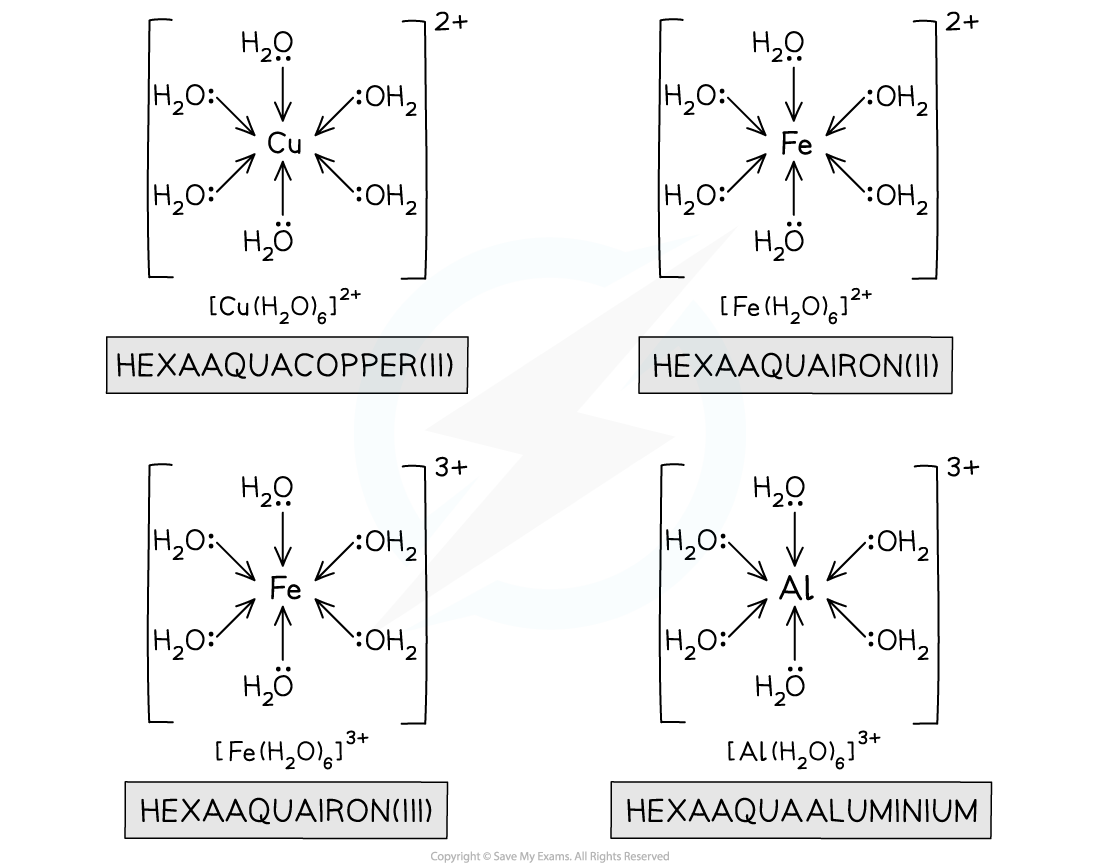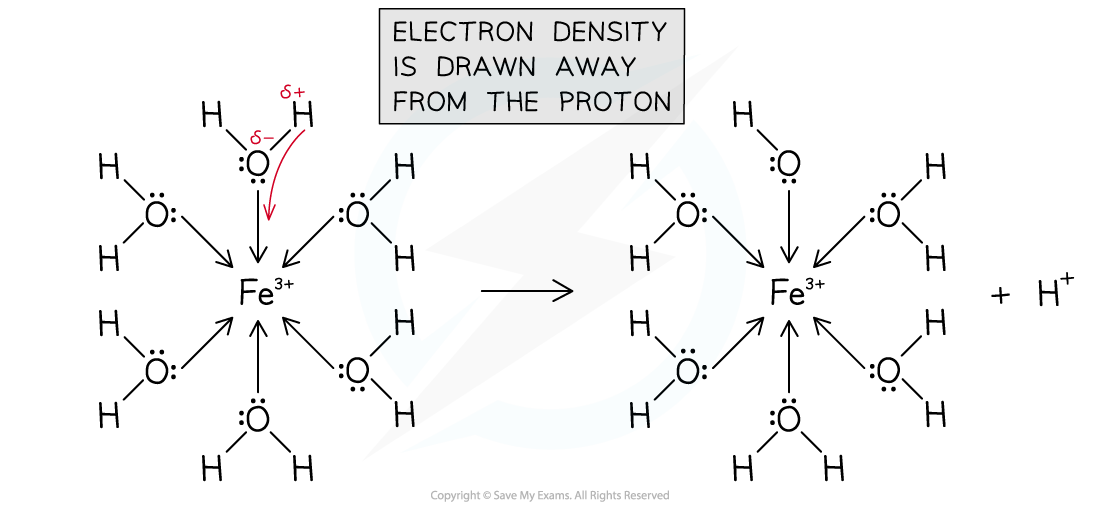# AQA A Level Chemistry复习笔记6.3.1 Metal-Aqua Ions

### Metal Aqua-Ions

• Transition metal salts that are readily soluble in water are forming metal-aqua complex ions when they dissolve in water
• When we show copper sulfate dissolving in water it is usually written as:

CuSO4 (s) + aq  → Cu2+ (aq)   + SO42- (aq)

• However, what we really mean by this is that copper(II) ions are forming the hexaaqucopper(II) ion

CuSO4 (s) + aq  → [Cu(H2O)6] 2+ (aq)   + SO42- (aq)

• The water molecules are ligands attached to the central transition metal cation by dative covalent bonding from the lone pairs on the oxygen molecules
• Iron(II) salts also form the hexaaqua complex ions:

Fe(NO3)2 (s) + aq  → [Fe(H2O)6] 2+ (aq)   + 2NO3- (aq)

• Two common +3 aqua ions you should know are iron(III) and aluminium:

Fe(NO3)3 (s) + aq  → [Fe(H2O)6] 3+ (aq)   + 3NO3- (aq)

Al2(SO4)3 (s) + aq  → 2[Al(H2O)6] 3+ (aq)   + 3SO42- (aq)Hexaaqua complex ions of +2 and +3 metal ions

#### Lewis Acids and Bases

• In the section of acids and bases we saw the Brønsted-Lowry definition of acids and bases

An acid is a substance which can behave as a proton donor

A base is a substance which can behave as a proton acceptor

• Gilbert Lewis is better known in the topic of bonding theory as the chemist whose name is given to electron dot structures of atoms, ions and molecules
• Lewis applied these structures to Brønsted-Lowry theory and realised that acid-base reactions can be interpreted in terms of electron pairs movements rather than in terms of proton transfer
• For example when ammonia reacts with a proton an electron pair moves from ammonia to the proton:

H+     +     NH3   →   NH4+The Lewis diagram shows that the base donates an electron pair to forming a dative covalent bond with the proton

• This lead to a new definition of acids and bases

A Lewis acid is a species which can bond by accepting a lone pair of electrons

A Lewis base is a species which can bond by donating a lone pair of electrons

• The bond formed is an example of a co-ordinate or dative covalent bond
• Following these definitions you should be able to see that in a metal-aqua complex ion:
• The metal is a Lewis acid
• The water is a Lewis base

• Although this definition of acids and bases is not required to be learned for the exam, you can see that it is a very useful concept for chemists when explaining movements of electrons pairs in a variety of situations

### Acidity in Metal-Aqua Ions

• You might imagine that salts of transition metal ions would be neutral in water
• However, ions of +3 aqua complexes are noticeably acidic compared to the +2 ions
• For example the pKa of [Fe(H2O)6] 3+ (aq) is 2.2 which is significantly more acidic than ethanoic acid which has a pKa of 4.8 (remember the lower the pKa the more acidic the species is)
• The reason for this is that +3 ions are smaller and therefore have a higher charge density than +2 ions• The higher charge density pulls the water molecules more strongly, which weakens the O-H bond and results in more dissociation, producing a more acidic solution
• We say that the metal ion polarises the water moleculesMetal(III) ions have a high charge density and polarise water molecules in the hexaaqua complexes

• This results in hydrogen ions (protons) splitting from the complexes creating acidic solutions

[Fe(H2O)6] 3+ (aq)   →    [Fe(H2O)5(OH)] 2+ (aq)  + H+ (aq)

• Notice that the loss of the proton results in the new pentaaqua complex having a +2 charge
• Another way to think of this is the left over hydroxide ion from splitting a water molecule is negatively charged and cancels one of the charges on the complex ion

• The reaction process can also be shown with the hydroxonium ion as the product:

[Fe(H2O)6] 3+ (aq) + H2O (l)   →    [Fe(H2O)5(OH)] 2+ (aq)  + H3O+ (aq)

• These reactions are sometime called deprotonation reactions and occur in several steps

[Fe(H2O)6] 3+ (aq)   →    [Fe(H2O)5(OH)] 2+ (aq)  + H+ (aq)

[Fe(H2O)5(OH)] 2+ (aq)   →    [Fe(H2O)4(OH)2] + (aq)  + H+ (aq)

• However, the third deprotonation does not usually occur without the presence of a base
• The base facilitates the removal of the third proton and results in the precipitation of the insoluble hydrated iron(III)hydroxide which appears as a foxy red precipitate:

[Fe(H2O)4(OH)2] + (aq)  + OH- →    Fe(H2O)3(OH)3 (s)  + H2O (l)

#### Exam Tip

The splitting of water molecules can be called a hydrolysis reaction